# Integration by substitution

## Summary and examples

In the topic Integration of standard funtions the primitive functions of a restricted number of functions are given.

The primitive functions of most functions cannot easily be calculated.
However, there are also special methods to calculate a primitive function. These methods can be quite complex, but here we focus on a special category, namely substitution.

The functions that we want to discuss now can be written as: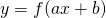met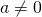If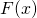is the primitive function of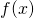then:is the primitive function of: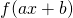We can verify whether this is correct by differentiating the primitive function, using the chain rule: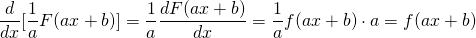And indeed, this result is correct.
The word substitution is used because the result above can also be calculated by the following substitution\: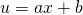and then differentiating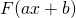: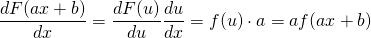The following examples show how the method of substitution works.

##### Example 1

Solve:The function: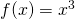has the primitive function: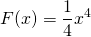We have for the integrand of the integral: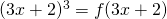and thus the primitive function of this function is: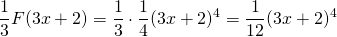and thus: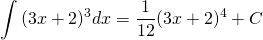The second method gives the same result.

Take: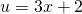Differentiating this function yields: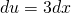or: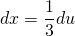If we subtitute these results in the original integral, we get: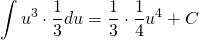If we 'transform back':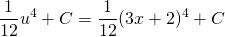It is a matter of personal preference which of the methods is chosen. In the following examples we will choose either method.

##### Example 2

Solve: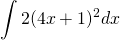The primitive of: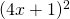is equal to: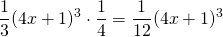and thus: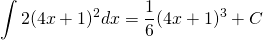##### Example 3

Solve: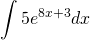We write: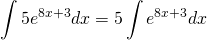and the primitive of: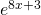is equal to: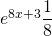So we can write: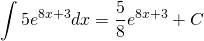##### Example 4

Solve: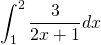We write this integral as:The primitive of:is: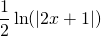and thus: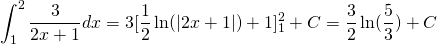##### Example 5

Solve: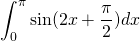The primitive of: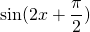is equal to: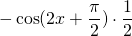and thus: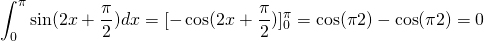0